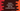# Python program to find the smallest divisor of a number## Smallest divisor of a number in Python :

This tutorial is to show you how we can find out the smallest divisor of a number in python. The number ‘y’ is called the divisor of a number ‘x’ if ‘x/y’ is 0. Our program will ask the user to enter a no. It will then find out the lowest divisor of that number.

If the number is 10, then its divisors are 1,2,5 and 10. We will ignore 1 and consider 2 as the smallest divisor for the number.

### Python program :

Let’s try to implement it in python :

``````#1
num = int(input("Enter a number : "))

#2
for i in range(2, num+1):
#3
if num % i == 0:
print ("The smallest divisor for {} is {}".format(num, i))
break``````

### Explanation :

The commented numbers in the above program denote the step numbers below :

1. Ask the user to enter a number. Read it by using the input() function. It will read the user input data as a string. Convert it by wrapping it with the int() function.
2. Run one for loop from 2 to the user input number.
3. For each number, check if we can divide the user input number by this number or not. We are using an if condition here. If the current number can divide the user input number, this will be the smallest divisor for that number. Print that number.

### Sample Outputs :

``````Enter a number : 13
The smallest divisor for 13 is 13

Enter a number : 14
The smallest divisor for 14 is 2

Enter a number : 100
The smallest divisor for 100 is 2``````

This program is available in Github.

### Conclusion :

We have learned how to find out the smallest divisor of a number in python. Try to run the program and drop one comment below if you have any queries.